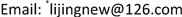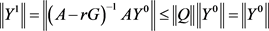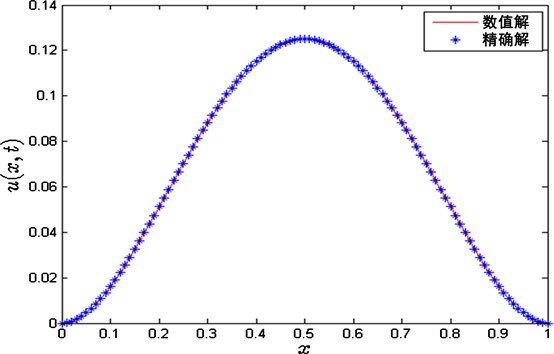﻿ 空间分布阶时间分数阶扩散方程的有限体积法 Finite Volume Method for a Space Distributed-Order Time-Fractional Diffusion Equation

Pure Mathematics
Vol. 09  No. 03 ( 2019 ), Article ID: 30297 , 11 pages
10.12677/PM.2019.93047

Finite Volume Method for a Space Distributed-Order Time-Fractional Diffusion Equation

Yingying Yang, Jing Li*

School of Mathematics and Statistics, Changsha University of Science and Technology, Changsha HunanReceived: Apr. 25th, 2019; accepted: May 5th, 2019; published: May 20th, 2019ABSTRACT

In this paper, the space distributed-order time-fractional diffusion equation is considered. We propose a finite volume method to solve the considered equation. Firstly, we use the mid-point quadrature rule to transform the space distributed-order term into a multi-term fractional term, and the multi-term fractional equation is discretized by the finite volume. For the time-fractional derivative, we apply the finite difference method. We prove that the iterative scheme is unconditionally stable and convergent. A numerical example is presented to verify the effectiveness of the proposed method.

Keywords:Space Distributed-Order Equation, Time-Fractional Diffusion Equation, Finite Volume Method, Stability and Convergence1. 引言

$\frac{{\partial }^{\beta }u\left(x,t\right)}{\partial {t}^{\beta }}={\int }_{1}^{2}P\left(\alpha \right)\frac{{\partial }^{\alpha }u\left(x,t\right)}{\partial {|x|}^{\alpha }}\text{d}\alpha +f\left(x,t\right),\text{\hspace{0.17em}}\left(x,t\right)\in \left(0,L\right)×\left(0,T\right)$ , (1)

$f\left(x,t\right)$ 是源项， $P\left(\alpha \right)$ 是满足下面条件的非负权函数：

$P\left(\alpha \right)\ge 0$ , $P\left(\alpha \right)\overline{)\equiv }0$ , $\alpha \in \left(1,2\right)$ , $0<{\int }_{1}^{2}P\left(\alpha \right)\text{d}\alpha <\infty$ .

l和K是正的常数， ${A}_{1}>0$${A}_{2}>0$$0<{\alpha }_{1}<{\alpha }_{2}\le 2$$\frac{{\partial }^{\beta }u\left(x,t\right)}{\partial {t}^{\beta }}$ 定义为Caputo分数阶导数：

$\frac{{\partial }^{\beta }u\left(x,t\right)}{\partial {t}^{\beta }}=\frac{1}{\Gamma \left(1-\beta \right)}{\int }_{0}^{t}\frac{\partial u\left(x,\xi \right)}{\partial \xi }\frac{\text{d}\xi }{\left(t-\xi \right)}，\text{\hspace{0.17em}}0<\beta <1$ .

$\frac{{\partial }^{\alpha }u\left(x,t\right)}{\partial {|x|}^{\alpha }}$ 是Riesz分数阶导数，定义为：

$\frac{{\partial }^{\alpha }u\left(x,t\right)}{\partial {|x|}^{\alpha }}=-\frac{1}{2\mathrm{cos}\left(\alpha \text{π}/2\right)}\left(\frac{{\partial }^{\alpha }u\left(x,t\right)}{\partial {x}^{\alpha }}+\frac{{\partial }^{\alpha }u\left(x,t\right)}{\partial {\left(-x\right)}^{\alpha }}\right),\text{\hspace{0.17em}}1<\alpha \le 2$ .

2. 有限体积法求解空间分布阶时间分数阶扩散方程的Crank-Nicolson迭代格式

${t}_{n}=n\tau$$t=0,\text{​}\text{ }1,2,\cdots ,N$${x}_{i}=ih$$i=0,1,2,\cdots ,M$ 离散区间 $\left[0,L\right]×\left[0,T\right]$ ，其中 $h=L/M$$\tau =T/N$

$\begin{array}{c}\frac{{\partial }^{\beta }u\left(x,{t}_{n}\right)}{\partial {t}^{\beta }}=\frac{1}{\Gamma \left(1-\beta \right)}\underset{j=0}{\overset{n-1}{\sum }}{\int }_{j\tau }^{\left(j+1\right)\tau }\frac{\partial u\left(x,\xi \right)}{\partial \xi }\frac{\text{d}\xi }{{\left({t}_{n}-\xi \right)}^{\beta }}\\ =\frac{1}{\Gamma \left(1-\beta \right)}\underset{j=0}{\overset{n-1}{\sum }}\frac{u\left(x,{t}_{j+1}\right)-u\left(x,{t}_{j}\right)}{\tau }{\int }_{j\tau }^{\left(j+1\right)\tau }\frac{\text{d}\xi }{{\left({t}_{n}-\xi \right)}^{\beta }}+ο\left({\tau }^{2-\beta }\right)\\ =\frac{{\tau }^{1-\beta }}{\Gamma \left(2-\beta \right)}\underset{j=0}{\overset{n-1}{\sum }}\frac{u\left(x,{t}_{n-j}\right)-u\left(x,{t}_{n-j-1}\right)}{\tau }\left[{\left(j+1\right)}^{1-\beta }-{j}^{1-\beta }\right]+ο\left({\tau }^{2-\beta }\right)\\ =a\left[u\left(x,{t}_{n}\right)-u\left(x,{t}_{n-1}\right)\right]+a\underset{j=1}{\overset{n-1}{\sum }}\left[u\left(x,{t}_{n-j}\right)-u\left(x,{t}_{n-j-1}\right)\right]{b}_{j}+ο\left({\tau }^{2-\beta }\right)\end{array}$ (4)

${\int }_{1}^{2}P\left(\alpha \right)\frac{{\partial }^{\alpha }u\left(x,t\right)}{\partial {|x|}^{\alpha }}\text{d}\alpha ={\int }_{{1}^{+}}^{2}P\left(\alpha \right)\frac{{\partial }^{\alpha }u\left(x,t\right)}{\partial {|x|}^{\alpha }}\text{d}\alpha$ . (5)

$\begin{array}{l}{\int }_{{1}^{+}}^{2}P\left(\alpha \right)\frac{{\partial }^{\alpha }u\left(x,t\right)}{\partial {|x|}^{\alpha }}\text{d}\alpha \\ =\underset{k=1}{\overset{S}{\sum }}P\left({\alpha }_{k}\right)\frac{{\partial }^{{\alpha }_{k}}u\left(x,t\right)}{\partial {|x|}^{{\alpha }_{k}}}\Delta {\xi }_{k}+ο\left({\sigma }^{2}\right)\end{array}$ . (6)

$\begin{array}{l}a\left[u\left(x,{t}_{n}\right)-u\left(x,{t}_{n-1}\right)\right]+a\underset{j=1}{\overset{n-1}{\sum }}{b}_{j}\left[u\left(x,{t}_{n-j}\right)-u\left(x,{t}_{n-j-1}\right)\right]\\ =\sigma \underset{k=1}{\overset{S}{\sum }}P\left({\alpha }_{k}\right)\frac{{\partial }^{{\alpha }_{k}}u\left(x,{t}_{n}\right)}{\partial {|x|}^{{\alpha }_{k}}}+f\left(x,{t}_{n}\right)+ο\left({\tau }^{2-\beta }+{\sigma }^{2}\right)\\ =\sigma \underset{k=1}{\overset{S}{\sum }}{a}_{k}P\left({\alpha }_{k}\right)\frac{\partial }{\partial x}\left[\frac{{\partial }^{{\gamma }_{k}}u\left(x,{t}_{n}\right)}{\partial {x}^{{\gamma }_{k}}}-\frac{{\partial }^{{\gamma }_{k}}u\left(x,{t}_{n}\right)}{\partial {\left(-x\right)}^{{\gamma }_{k}}}\right]+f\left(x,{t}_{n}\right)+ο\left({\tau }^{2-\beta }+{\sigma }^{2}\right)\end{array}$ (7)

$\begin{array}{l}a{\int }_{{x}_{i-\frac{1}{2}}}^{{x}_{i+\frac{1}{2}}}u\left(x,{t}_{n}\right)\text{d}x-\sigma \underset{k=1}{\overset{S}{\sum }}{a}_{k}P\left({\alpha }_{k}\right){\left[\frac{{\partial }^{{\gamma }_{k}}u\left(x,{t}_{n}\right)}{\partial {x}^{{\gamma }_{k}}}-\frac{{\partial }^{{\gamma }_{k}}u\left(x,{t}_{n}\right)}{\partial {\left(-x\right)}^{{\gamma }_{k}}}\right]}_{{x}_{i-\frac{1}{2}}}^{{x}_{i+\frac{1}{2}}}\\ =a{\int }_{{x}_{i-\frac{1}{2}}}^{{x}_{i+\frac{1}{2}}}u\left(x,{t}_{n-1}\right)\text{d}x-a\underset{j=1}{\overset{n-1}{\sum }}{b}_{j}{\int }_{{x}_{i-\frac{1}{2}}}^{{x}_{i+\frac{1}{2}}}\left[u\left(x,{t}_{n-j}\right)-u\left(x,{t}_{n-j-1}\right)\right]\text{d}x+{\int }_{{x}_{i-\frac{1}{2}}}^{{x}_{i+\frac{1}{2}}}f\left(x,{t}_{n}\right)\text{d}x+ο\left(h\left({\tau }^{2-\beta }+\sigma \right)\right)\end{array}$ (8)

${\varphi }_{i},0\le i\le M$${V}_{h}$ 的节点基函数。 ${\varphi }_{0},{\varphi }_{1},\cdots ,{\varphi }_{M}$ 表示为下面形式：

${\varphi }_{i}\left(x\right)=\left\{\begin{array}{l}\frac{x-{x}_{i-1}}{h},\text{ }x\in \left[{x}_{i-1},{x}_{i}\right]\\ \frac{{x}_{i+1}-x}{h},\text{ }x\in \left[{x}_{i},{x}_{i+1}\right],\text{ }i=1,2,\cdots ,M-1\\ 0,\text{\hspace{0.17em}}\text{ }\text{ }\text{ }\text{\hspace{0.17em}}其它\end{array}$${\varphi }_{0}\left(x\right)=\left\{\begin{array}{l}\frac{{x}_{1}-x}{h},\text{ }x\in \left[{x}_{0},{x}_{1}\right]\\ 0,\text{\hspace{0.17em}}\text{ }\text{ }\text{ }\text{\hspace{0.17em}}其它\end{array}$${\varphi }_{M}\left(x\right)=\left\{\begin{array}{l}\frac{x-{x}_{M-1}}{h},\text{ }x\in \left[{x}_{M-1},{x}_{M}\right]\\ 0,\text{\hspace{0.17em}}\text{ }\text{ }\text{ }\text{\hspace{0.17em}}\text{\hspace{0.17em}}\text{\hspace{0.17em}}\text{\hspace{0.17em}}其它\end{array}$

$\begin{array}{l}a\underset{m=1}{\overset{M-1}{\sum }}{u}_{m}^{n}{\int }_{{x}_{i-\frac{1}{2}}}^{{x}_{i+\frac{1}{2}}}{\varphi }_{m}\left(x\right)\text{d}x-\sigma \underset{m=1}{\overset{M-1}{\sum }}{u}_{m}^{n}\underset{k=1}{\overset{S}{\sum }}{a}_{k}P\left({\alpha }_{k}\right){\left[\frac{{\partial }^{{\gamma }_{k}}{\varphi }_{m}\left(x\right)}{\partial {x}^{{\gamma }_{k}}}-\frac{{\partial }^{{\gamma }_{k}}{\varphi }_{m}\left(x\right)}{\partial {\left(-x\right)}^{{\gamma }_{k}}}\right]}_{{x}_{i-\frac{1}{2}}}^{{x}_{i+\frac{1}{2}}}\\ =a\underset{m=1}{\overset{M-1}{\sum }}{u}_{m}^{n-1}{\int }_{{x}_{i-\frac{1}{2}}}^{{x}_{i+\frac{1}{2}}}{\varphi }_{m}\left(x\right)\text{d}x-a\underset{m=1}{\overset{M-1}{\sum }}\underset{j=1}{\overset{n-1}{\sum }}{b}_{j}{u}_{m}^{n-j}{\int }_{{x}_{i-\frac{1}{2}}}^{{x}_{i+\frac{1}{2}}}{\varphi }_{m}\left(x\right)\text{d}x+a\underset{m=1}{\overset{M-1}{\sum }}\underset{j=1}{\overset{n-1}{\sum }}{b}_{j}{u}_{m}^{n-j-1}{\int }_{{x}_{i-\frac{1}{2}}}^{{x}_{i+\frac{1}{2}}}{\varphi }_{m}\left(x\right)\text{d}x+{\int }_{{x}_{i-\frac{1}{2}}}^{{x}_{i+\frac{1}{2}}}f\left(x,{t}_{n}\right)\text{d}x\end{array}$ (9)

${\int }_{{x}_{i-\frac{1}{2}}}^{{x}_{i+\frac{1}{2}}}{\varphi }_{m}\left(x\right)\text{d}x=\left\{\begin{array}{l}h/8,\text{ }\text{\hspace{0.17em}}|i-m|=1\\ 3h/4,\text{ }i=m\\ 0,\text{ }\text{ }\text{\hspace{0.17em}}\text{\hspace{0.17em}}其它\end{array}$

$\frac{{\partial }^{{\gamma }_{k}}{\varphi }_{m}\left({x}_{i+1/2}\right)}{\partial {x}^{{\gamma }_{k}}}=\frac{1}{\Gamma \left(2-{\gamma }_{k}\right){h}^{{\gamma }_{k}}}\left\{\begin{array}{l}0,\text{ }\text{ }\text{ }\text{ }\text{ }\text{\hspace{0.17em}}\text{\hspace{0.17em}}\text{\hspace{0.17em}}m>i+1\\ {2}^{{\gamma }_{k}-1},\text{ }\text{ }\text{ }\text{ }\text{\hspace{0.17em}}m=i+1\\ {\left(3/2\right)}^{1-{\gamma }_{k}}-{2}^{{\gamma }_{k}},\text{\hspace{0.17em}}\text{\hspace{0.17em}}m=i\\ {c}_{i-m+1}^{k},\text{ }\text{ }\text{ }\text{\hspace{0.17em}}\text{\hspace{0.17em}}\text{\hspace{0.17em}}\text{ }m

$\frac{{\partial }^{{\gamma }_{k}}{\varphi }_{m}\left({x}_{i-1/2}\right)}{\partial {x}^{{\gamma }_{k}}}=\frac{1}{\Gamma \left(2-{\gamma }_{k}\right){h}^{{\gamma }_{k}}}\left\{\begin{array}{l}0,\text{ }\text{ }\text{ }\text{ }\text{ }\text{\hspace{0.17em}}\text{\hspace{0.17em}}m>i\\ {2}^{{\gamma }_{k}-1},\text{ }\text{ }\text{ }\text{ }\text{ }\text{ }m=i\\ {\left(3/2\right)}^{1-{\gamma }_{k}}-{2}^{{\gamma }_{k}},\text{ }\text{ }\text{ }\text{ }\text{ }m=i-1\\ {c}_{i-m}^{k},\text{ }\text{ }\text{ }\text{\hspace{0.17em}}\text{\hspace{0.17em}}\text{\hspace{0.17em}}\text{\hspace{0.17em}}\text{ }\text{ }m

$\frac{{\partial }^{{\gamma }_{k}}{\varphi }_{m}\left({x}_{i+1/2}\right)}{\partial {\left(-x\right)}^{{\gamma }_{k}}}=\frac{1}{\Gamma \left(2-{\gamma }_{k}\right){h}^{{\gamma }_{k}}}\left\{\begin{array}{l}{c}_{m-i}^{k},\text{ }\text{ }\text{ }\text{ }\text{\hspace{0.17em}}m>i+1\\ {\left(3/2\right)}^{1-{\gamma }_{k}}-{2}^{{\gamma }_{k}},m=i-1\\ {2}^{{\gamma }_{k}-1},\text{ }\text{ }\text{ }\text{ }m=i\\ 0,\text{ }\text{ }\text{ }\text{\hspace{0.17em}}\text{\hspace{0.17em}}\text{\hspace{0.17em}}\text{\hspace{0.17em}}\text{\hspace{0.17em}}\text{ }m

$\frac{{\partial }^{{\gamma }_{k}}{\varphi }_{m}\left({x}_{i-1/2}\right)}{\partial {\left(-x\right)}^{{\gamma }_{k}}}=\frac{1}{\Gamma \left(2-{\gamma }_{k}\right){h}^{{\gamma }_{k}}}\left\{\begin{array}{l}{c}_{m-i+1}^{k},\text{ }\text{ }\text{ }\text{\hspace{0.17em}}\text{​}\text{\hspace{0.17em}}\text{ }m>i\\ {\left(3/2\right)}^{1-{\gamma }_{k}}-{2}^{{\gamma }_{k}},\text{\hspace{0.17em}}m=i\\ {2}^{{\gamma }_{k}-1},\text{ }\text{ }\text{ }\text{ }m=i-1\\ 0,\text{ }\text{ }\text{ }\text{\hspace{0.17em}}\text{\hspace{0.17em}}\text{\hspace{0.17em}}\text{\hspace{0.17em}}\text{\hspace{0.17em}}\text{ }m

${G}_{1,im}=\sigma \underset{k=1}{\overset{S}{\sum }}{a}_{k}P\left({\alpha }_{k}\right)\left[\frac{{\partial }^{{\gamma }_{k}}{\varphi }_{m}\left({x}_{i+1/2}\right)}{\partial {x}^{{\gamma }_{k}}}-\frac{{\partial }^{{\gamma }_{k}}{\varphi }_{m}\left({x}_{i-1/2}\right)}{\partial {x}^{{\gamma }_{k}}}\right]$ , ${G}_{2,im}=\sigma \underset{k=1}{\overset{S}{\sum }}{a}_{k}P\left({\alpha }_{k}\right)\left[\frac{{\partial }^{{\gamma }_{k}}{\varphi }_{m}\left({x}_{i+1/2}\right)}{\partial {\left(-x\right)}^{{\gamma }_{k}}}-\frac{{\partial }^{{\gamma }_{k}}{\varphi }_{m}\left({x}_{i-1/2}\right)}{\partial {\left(-x\right)}^{{\gamma }_{k}}}\right]$ .

$a\frac{h}{8}\left({u}_{i-1}^{1}+6{u}_{i}^{1}+{u}_{i+1}^{1}\right)-\underset{m=1}{\overset{M-1}{\sum }}{u}_{m}^{1}{G}_{im}=a\frac{h}{8}\left({u}_{i-1}^{0}+6{u}_{i}^{0}+{u}_{i+1}^{0}\right)+{\left({F}^{1}\right)}_{i}$ , (10)

$n>1$ 时，(11)

$\left\{\begin{array}{l}\left(A-rG\right){U}^{1}=A{U}^{0}+rF{}^{1},\text{ }\text{ }\text{ }\text{ }\text{ }\text{ }\text{ }\text{ }\text{ }\text{ }\text{ }\text{ }\text{ }\text{ }\text{\hspace{0.17em}}\text{\hspace{0.17em}}\text{\hspace{0.17em}}\text{\hspace{0.17em}}\text{\hspace{0.17em}}\text{ }n=1\\ \left(A-rG\right){U}^{n}=A\left({d}_{1}{U}^{n-1}+{d}_{2}{U}^{n-2}+\cdots +{d}_{n-1}{U}^{1}+{b}_{n-1}{U}^{0}\right)+rF{}^{n},\text{\hspace{0.17em}}\text{\hspace{0.17em}}\text{ }n>1\end{array}$ (12)

3. 迭代方法的稳定性和收敛性

$|{G}_{ii}|>\underset{m=1,m\ne i}{\overset{M-1}{\sum }}|{G}_{im}|,i=1,2,\cdots ,M-1$ ，则G是严格对角占优的。

${B}_{im}=\left\{\begin{array}{l}r\sigma \underset{k=1}{\overset{S}{\sum }}\frac{{a}_{k}P\left({\alpha }_{k}\right)}{\Gamma \left(2-{\gamma }_{k}\right){h}^{{\gamma }_{k}}}\left({c}_{m-i}^{k}-{c}_{m-i+1}^{k}\right),\text{ }\text{ }\text{ }\text{ }\text{ }\text{ }\text{ }\text{ }\text{\hspace{0.17em}}\text{\hspace{0.17em}}m>i+1\\ \frac{h}{8}-r\sigma \underset{k=1}{\overset{S}{\sum }}\frac{{a}_{k}P\left({\alpha }_{k}\right)}{\Gamma \left(2-{\gamma }_{k}\right){h}^{{\gamma }_{k}}}\left[3{\left(\frac{1}{2}\right)}^{1-{\gamma }_{k}}-{\left(\frac{3}{2}\right)}^{1-{\gamma }_{k}}+{c}_{2}^{k}\right]，\text{ }\text{ }\text{\hspace{0.17em}}m=i+1\\ \frac{3h}{4}-r\sigma \underset{k=1}{\overset{S}{\sum }}\frac{{a}_{k}P\left({\alpha }_{k}\right)}{\Gamma \left(2-{\gamma }_{k}\right){h}^{{\gamma }_{k}}}2\left[{\left(\frac{3}{2}\right)}^{1-{\gamma }_{k}}-3{\left(\frac{1}{2}\right)}^{1-{\gamma }_{k}}\right],\text{ }\text{ }\text{ }m=i\\ \frac{h}{8}-r\sigma \underset{k=1}{\overset{S}{\sum }}\frac{{a}_{k}P\left({\alpha }_{k}\right)}{\Gamma \left(2-{\gamma }_{k}\right){h}^{{\gamma }_{k}}}\left[3{\left(\frac{1}{2}\right)}^{1-{\gamma }_{k}}-{\left(\frac{3}{2}\right)}^{1-{\gamma }_{k}}+{c}_{2}^{k}\right],\text{ }\text{ }\text{\hspace{0.17em}}m=i-1\\ r\sigma \underset{k=1}{\overset{S}{\sum }}\frac{{a}_{k}P\left({\alpha }_{k}\right)}{\Gamma \left(2-{\gamma }_{k}\right){h}^{{\gamma }_{k}}}\left({c}_{i-m}^{k}-{c}_{i-m+1}^{k}\right),\text{ }\text{ }\text{ }\text{ }\text{ }\text{ }\text{ }\text{ }\text{\hspace{0.17em}}\text{\hspace{0.17em}}m

$|{D}_{ii}|>\underset{m=1,m\ne i}{\overset{M-1}{\sum }}|{D}_{im}|,\text{\hspace{0.17em}}i=1,2,\cdots ,M-1$ .

${D}_{im}=\left\{\begin{array}{l}\lambda r\sigma \underset{k=1}{\overset{S}{\sum }}\frac{{a}_{k}P\left({\alpha }_{k}\right)}{\Gamma \left(2-{\gamma }_{k}\right){h}^{{\gamma }_{k}}}\left({c}_{m-i}^{k}-{c}_{m-i+1}^{k}\right),\text{ }\text{ }\text{ }\text{ }\text{ }\text{ }\text{ }\text{ }\text{\hspace{0.17em}}\text{\hspace{0.17em}}\text{ }\text{ }\text{\hspace{0.17em}}\text{\hspace{0.17em}}\text{\hspace{0.17em}}m>i+1\\ \left(\lambda -1\right)\frac{h}{8}-\lambda r\sigma \underset{k=1}{\overset{S}{\sum }}\frac{{a}_{k}P\left({\alpha }_{k}\right)}{\Gamma \left(2-{\gamma }_{k}\right){h}^{{\gamma }_{k}}}\left[3{\left(\frac{1}{2}\right)}^{1-{\gamma }_{k}}-{\left(\frac{3}{2}\right)}^{1-{\gamma }_{k}}+{c}_{2}^{k}\right],\text{ }\text{ }\text{\hspace{0.17em}}m=i+1\\ \left(\lambda -1\right)\frac{3h}{4}-\lambda r\sigma \underset{k=1}{\overset{S}{\sum }}\frac{{a}_{k}P\left({\alpha }_{k}\right)}{\Gamma \left(2-{\gamma }_{k}\right){h}^{{\gamma }_{k}}}2\left[{\left(\frac{3}{2}\right)}^{1-{\gamma }_{k}}-3{\left(\frac{1}{2}\right)}^{1-{\gamma }_{k}}\right],\text{ }\text{ }\text{ }m=i\\ \left(\lambda -1\right)\frac{h}{8}-\lambda r\sigma \underset{k=1}{\overset{S}{\sum }}\frac{{a}_{k}P\left({\alpha }_{k}\right)}{\Gamma \left(2-{\gamma }_{k}\right){h}^{{\gamma }_{k}}}\left[3{\left(\frac{1}{2}\right)}^{1-{\gamma }_{k}}-{\left(\frac{3}{2}\right)}^{1-{\gamma }_{k}}+{c}_{2}^{k}\right],\text{ }\text{ }\text{\hspace{0.17em}}m=i-1\\ \lambda r\sigma \underset{k=1}{\overset{S}{\sum }}\frac{{a}_{k}P\left({\alpha }_{k}\right)}{\Gamma \left(2-{\gamma }_{k}\right){h}^{{\gamma }_{k}}}\left({c}_{i-m}^{k}-{c}_{i-m+1}^{k}\right),\text{ }\text{ }\text{ }\text{ }\text{ }\text{ }\text{ }\text{ }\text{\hspace{0.17em}}\text{\hspace{0.17em}}\text{ }\text{ }\text{\hspace{0.17em}}\text{\hspace{0.17em}}m

$n=1$ 时，将 ${\epsilon }_{i}^{n}={\stackrel{˜}{u}}_{i}^{n}-{u}_{i}^{n}$ 代入(12)，得到 ${Y}^{1}={\left(A-rG\right)}^{-1}A{Y}^{0}$ 。令 $Q={\left(A-rG\right)}^{-1}A$ ，根据定理4，我们知道 $\rho \left(Q\right)<1$ ，则存在向量范数和诱导矩阵范数 $‖\text{\hspace{0.17em}}.\text{\hspace{0.17em}}‖$ ，使 $‖Q‖<1$ 。使用以上范数，得到：.

$\begin{array}{c}‖{Y}^{k}‖=‖{\left(A-rG\right)}^{-1}A\left({d}_{1}{Y}^{k-1}+{d}_{2}{Y}^{k-2}+\cdots +{d}_{k-1}{Y}^{1}+{b}_{k-1}{Y}^{0}\right)‖\\ \le ‖\text{\hspace{0.17em}}Q\text{\hspace{0.17em}}‖\left({d}_{1}+{d}_{2}+\cdots +{d}_{k-1}+{b}_{k-1}\right)‖{Y}^{0}‖\\ \le \left({d}_{1}+{d}_{2}+\cdots +{d}_{k-1}+{b}_{k-1}\right)‖{Y}^{0}‖\\ =\left(1-{b}_{k-1}+{b}_{k-1}\right)‖{Y}^{0}‖\\ =‖{Y}^{0}‖\end{array}$

$‖{u}^{n}-{U}^{n}‖\le C\left({\tau }^{2-\beta }+{\sigma }^{2}+{h}^{2}\right)$ .

$\left\{\begin{array}{l}\frac{h}{8}\left({e}_{i-1}^{1}+6{e}_{i}^{1}+{e}_{i+1}^{1}\right)-r\underset{m=1}{\overset{M-1}{\sum }}{e}_{m}^{1}{G}_{im}=\frac{h}{8}\left({e}_{i-1}^{0}+6{e}_{i}^{0}+{e}_{i+1}^{0}\right)+rο\left(h\left({\tau }^{2-\beta }+{\sigma }^{2}+{h}^{2}\right)\right),\text{ }\text{ }\text{ }\text{ }\text{ }\text{ }\text{ }\text{\hspace{0.17em}}n=1\\ \frac{h}{8}\left({e}_{i-1}^{n}+6{e}_{i}^{n}+{e}_{i+1}^{n}\right)-r\underset{m=1}{\overset{M-1}{\sum }}{e}_{m}^{n}{G}_{im}=\left(1-{b}_{1}\right)\frac{h}{8}\left({e}_{i-1}^{n-1}+6{e}_{i}^{n-1}+{e}_{i+1}^{n-1}\right)\\ \text{ }+\underset{j=1}{\overset{n-2}{\sum }}\frac{h}{8}\left({e}_{i-1}^{n-j-1}+6{e}_{i}^{n-j-1}+{e}_{i+1}^{n-j-1}\right)\left({b}_{j}-{b}_{j+1}\right)+\frac{h}{8}\left({e}_{i-1}^{0}+6{e}_{i}^{0}+{e}_{i+1}^{0}\right){b}_{n-1}+rο\left(h\left({\tau }^{2-\beta }+{\sigma }^{2}+{h}^{2}\right)\right),\text{\hspace{0.17em}}\text{\hspace{0.17em}}n>1\end{array}$

$\left\{\begin{array}{l}\left(A-rG\right){E}^{1}=A{E}^{0}+rο\left(h\left({\tau }^{2-\beta }+{\sigma }^{2}+{h}^{2}\right)\right)\chi ,\text{ }\text{ }\text{ }\text{ }\text{ }\text{ }\text{ }\text{ }\text{ }\text{ }\text{ }\text{ }\text{ }\text{ }\text{\hspace{0.17em}}\text{\hspace{0.17em}}\text{\hspace{0.17em}}\text{\hspace{0.17em}}\text{\hspace{0.17em}}\text{ }n=1\\ \left(A-rG\right){E}^{n}=A\left({d}_{1}{E}^{n-1}+{d}_{2}{E}^{n-2}+\cdots +{d}_{n-1}{E}^{1}+{b}_{n-1}{E}^{0}\right)+rο\left(h\left({\tau }^{2-\beta }+{\sigma }^{2}+{h}^{2}\right)\right)\chi ,\text{\hspace{0.17em}}\text{\hspace{0.17em}}\text{ }\text{\hspace{0.17em}}n>1\end{array}$

$\chi ={\left(1,\text{\hspace{0.17em}}1,\text{\hspace{0.17em}}\cdots ,\text{\hspace{0.17em}}1\right)}^{\text{T}},\text{\hspace{0.17em}}{E}^{n}={\left({e}_{1}^{n},\text{\hspace{0.17em}}{e}_{2}^{n},\text{\hspace{0.17em}}\cdots ,\text{\hspace{0.17em}}{e}_{M-1}^{n}\right)}^{\text{T}},\text{\hspace{0.17em}}Q={\left(A-rG\right)}^{-1}A,\text{\hspace{0.17em}}B=A-rG$ .

$n=1$ 时，得到

${E}^{1}=Q{E}^{0}+r{\left(A-rG\right)}^{-1}ο\left(h\left({\tau }^{2-\beta }+{\sigma }^{2}+{h}^{2}\right)\right)\chi =r{B}^{-1}ο\left(h\left({\tau }^{2-\beta }+{\sigma }^{2}+{h}^{2}\right)\right)\chi$ .

$‖{E}^{1}‖=‖r{B}^{-1}ο\left(h\left({\tau }^{2-\beta }+{\sigma }^{2}+{h}^{2}\right)\right)\chi ‖\le ο\left({\tau }^{\beta }\left({\tau }^{2-\beta }+{\sigma }^{2}+{h}^{2}\right)\right)$ .

$‖{E}^{k-1}‖\le ο\left({\tau }^{\beta }\left({\tau }^{2-\beta }+{\sigma }^{2}+{h}^{2}\right)\right)$ .

$\begin{array}{c}‖{E}^{k}‖=‖Q\left({d}_{1}{E}^{k-1}+{d}_{2}{E}^{k-2}+\cdots +{d}_{k-1}{E}^{1}+{b}_{k-1}{E}^{0}\right)+r{B}^{-1}ο\left(h\left({\tau }^{2-\beta }+{\sigma }^{2}+{h}^{2}\right)\right)\chi ‖\\ \le ‖Q‖\left({d}_{1}+{d}_{2}+\cdots +{d}_{k-1}+{b}_{k-1}\right)ο\left({\tau }^{\beta }\left({\tau }^{2-\beta }+{\sigma }^{2}+{h}^{2}\right)\right)+ο\left({\tau }^{\beta }\left({\tau }^{2-\beta }+{\sigma }^{2}+{h}^{2}\right)\right)\\ \le \left(1-b{}_{k-1}+{b}_{k-1}\right)ο\left({\tau }^{\beta }\left({\tau }^{2-\beta }+{\sigma }^{2}+{h}^{2}\right)\right)\\ =ο\left({\tau }^{\beta }\left({\tau }^{2-\beta }+{\sigma }^{2}+{h}^{2}\right)\right)\end{array}$

4. 数值例子

$\frac{{\partial }^{\beta }u\left(x,t\right)}{\partial {t}^{\beta }}={\int }_{1}^{2}P\left(\alpha \right)\frac{{\partial }^{\alpha }u\left(x,t\right)}{\partial {|x|}^{\alpha }}\text{d}\alpha +f\left(x,t\right),\text{\hspace{0.17em}}\left(x,t\right)\in \left(0,1\right)×\left(0,1\right]$ ,

$P\left(\alpha \right)=-2\Gamma \left(5-\alpha \right)\mathrm{cos}\left(\frac{\text{π}\alpha }{2}\right)$ ,

$\begin{array}{c}f\left(x,t\right)=\frac{2}{\Gamma \left(3-\beta \right)}{t}^{2-\beta }{x}^{2}{\left(1-x\right)}^{2}-{\int }_{1}^{2}P\left(\alpha \right)\frac{{\partial }^{\alpha }u\left(x,t\right)}{\partial {|x|}^{\alpha }}\text{d}\alpha \\ =\frac{2}{\Gamma \left(3-\beta \right)}{t}^{2-\beta }{x}^{2}{\left(1-x\right)}^{2}-\left({t}^{2}+1\right)\left[R\left(x\right)+R\left(1-x\right)\right]\end{array}$ ,

$\begin{array}{c}R\left(x\right)=\Gamma \left(5\right)\frac{1}{\mathrm{ln}x}\left({x}^{3}-{x}^{2}\right)-2\Gamma \left(4\right)\left[\frac{1}{\mathrm{ln}x}\left(3{x}^{2}-2x\right)-\frac{1}{{\left(\mathrm{ln}x\right)}^{2}}\left({x}^{2}-x\right)\right]\\ \text{\hspace{0.17em}}\text{\hspace{0.17em}}+\Gamma \left(3\right)\frac{1}{\mathrm{ln}x}\left[6x-2-\frac{5x}{\mathrm{ln}x}+\frac{3}{\mathrm{ln}x}+\frac{2x}{{\left(\mathrm{ln}x\right)}^{2}}-\frac{2}{{\left(\mathrm{ln}x\right)}^{2}}\right]\end{array}$Figure 1. Exact solution and numerical solution with $\sigma =\tau =h=1/100$ at $t=1.0$ and $\beta =0.7$Table 1. The error and the convergence order of σ for τ = h = 1 / 1000 at t = 1 and β = 0.7Table 2. The error and the convergence order of h for τ = σ = 1 / 500 at and β = 0.7Table 3. The error and the convergence order of τ for h = σ = 1 / 400 at t = 1 and β = 0.7

5. 结论

Finite Volume Method for a Space Distributed-Order Time-Fractional Diffusion Equation[J]. 理论数学, 2019, 09(03): 351-361. https://doi.org/10.12677/PM.2019.93047

1. 1. Caputo, M. (1995) Mean Fractional-Order-Derivatives Differential Equations and Filters. Annali dell Universita di Ferrara, 41, 73-84.

2. 2. Hu, X., Liu, F., Turner, I. and Anh, V. (2016) An Implicit Numerical Method of a New Time Distributed-Order and Two-Sided Space-Fractional Advection-Dispersion Equation. Numerical Algorithms, 72, 393-407.
https://doi.org/10.1007/s11075-015-0051-1

3. 3. Bu, W., Xiao, A. and Zeng, W. (2017) Finite Difference/Finite Element Methods for Distributed-Order Time Fractional Diffusion Equations. Journal of Scientific Computing, 72, 422-441.
https://doi.org/10.1007/s10915-017-0360-8

4. 4. Li, Z., Luchko, Y. and Yamamoto, M. (2017) Analyticity of Solutions to a Distributed Order Time-Fractional Diffusion Equation and Its Application to an Inverse Problem. Computers and Mathematics with Applications, 73, 1041-1052.
https://doi.org/10.1016/j.camwa.2016.06.030

5. 5. Liu, Q., Mu, S., Liu, Q., Liu, B., Bi, X., Zhuang, P. and Li, B. (2018) An RBF Based Meshless Method for the Distributed Order Time Fractional Advection-Diffusion Equation. Engineering Analysis with Boundary Elements, 96, 55-63.
https://doi.org/10.1016/j.enganabound.2018.08.007

6. 6. Li, J., Liu, F., Feng, L. and Turner, I. (2017) A Novel Finite Volume Method for the Riesz Space Distributed-Order Advection Diffusion Equation. Applied Mathematical Modelling, 46, 536-553.
https://doi.org/10.1016/j.apm.2017.01.065

7. 7. Jia, J. and Wang, H. (2018) A Fast Finite Difference Method for Distribut-ed-Order Space-Fractional Partial Differential Equations on Convex Domains. Computers and Mathematics with Applications, 75, 2031-2043.
https://doi.org/10.1016/j.camwa.2017.09.003

8. 8. Zhang, Y. and Meerschaert, M. (2008) Particle Tracking for Time Fractional Diffusion. Physical Review E, 78, 1-7.

9. 9. Soklov, I.M., Chechkin, A.V. and Klafter, J. (2004) Distributed-Order Fractional Kinetics. Acta Physica Polonica B, 35, 1323-1341.

10. 10. Lin, Y. and Xu, C. (2007) Finite Difference/Spectral Approximations for the Time-Fractional Diffusion Equation. Journal of Computational Physics, 225, 1533-1552.
https://doi.org/10.1016/j.jcp.2007.02.001

11. 11. Li, J., Liu, F., Feng, L. and Turner, I. (2017) A Novel Finite Volume Method for the Riesz Space Distributed-Order Diffusion Equation. Computers and Mathematics with Applications, 74, 772-783.
https://doi.org/10.1016/j.camwa.2017.05.017

12. 12. Shen, S., Liu, F., Anh, V. and Turner, I. (2006) Detailed Analysis of a Con-servative Difference Approximation for the Time Fractional Diffusion Equation. Journal of Computational and Applied Mathematics, 22, 1-19.
https://doi.org/10.1007/bf02832034

13. 13. Feng, L.B., Zhuang, P., Liu, F. and Turner, I. (2015) Stability and Convergence of a New Finite Volume Method for a Two-Sided Space-Fractional Diffusion Equation. Applied Mathematics and Computation, 257, 52-65.
https://doi.org/10.1016/j.amc.2014.12.060

NOTES

*通讯作者。